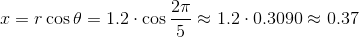# High School Math : Understanding Polar Coordinates

## Example Questions

### Example Question #5 : Calculus Ii — Integrals

The polar coordinates of a point are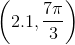. Give its-coordinate in the rectangular coordinate system (nearest hundredth).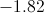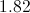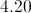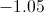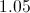Explanation:

Given the polar coordinates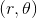, the-coordinate is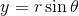.  We can find this coordinate by substituting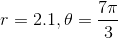: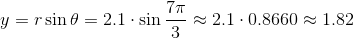### Example Question #2 : Parametric, Polar, And Vector

The polar coordinates of a point are. Give its-coordinate in the rectangular coordinate system (nearest hundredth).Explanation:

Given the polar coordinates, the-coordinate is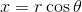. We can find this coordinate by substituting: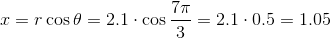### Example Question #1 : Parametric, Polar, And Vector

The polar coordinates of a point are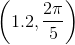. Give its-coordinate in the rectangular coordinate system (nearest hundredth).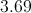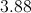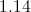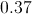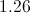Explanation:

Given the polar coordinates, the-coordinate is. We can find this coordinate by substituting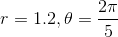:### Example Question #6 : Calculus Ii — Integrals

The polar coordinates of a point are. Give its-coordinate in the rectangular coordinate system (nearest hundredth).Given the polar coordinates, the-coordinate is. We can find this coordinate by substituting: